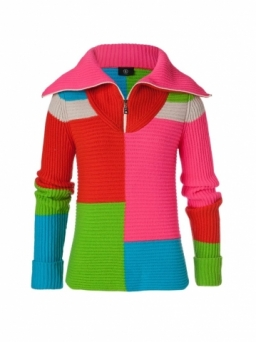# Sweaters 8377

The store earned CZK 1,950 for 13 jackets and five sweaters of the same size and quality. The sweater was 350 CZK cheaper than the jacket. Find the price of the jacket and sweater.

b =  1200
s =  850

### Step-by-step explanation:

13b+5s = 19850
s = b - 350

13·b+5·s = 19850
s = b - 350

13b+5s = 19850
b-s = 350

Row 2 - 1/13 · Row 1 → Row 2
13b+5s = 19850
-1.38s = -1176.92

s = -1176.92307692/-1.38461538 = 850
b = 19850-5s/13 = 19850-5 · 850/13 = 1200

b = 1200
s = 850

Our linear equations calculator calculates it.Did you find an error or inaccuracy? Feel free to write us. Thank you!

Tips for related online calculators
Do you have a system of equations and looking for calculator system of linear equations?